Complex Numbers & Quadratic Equations- 2

# Complex Numbers & Quadratic Equations- 2 - Commerce

Test Description

## 25 Questions MCQ Test Mathematics (Maths) Class 11 - Complex Numbers & Quadratic Equations- 2

Complex Numbers & Quadratic Equations- 2 for Commerce 2023 is part of Mathematics (Maths) Class 11 preparation. The Complex Numbers & Quadratic Equations- 2 questions and answers have been prepared according to the Commerce exam syllabus.The Complex Numbers & Quadratic Equations- 2 MCQs are made for Commerce 2023 Exam. Find important definitions, questions, notes, meanings, examples, exercises, MCQs and online tests for Complex Numbers & Quadratic Equations- 2 below.
Solutions of Complex Numbers & Quadratic Equations- 2 questions in English are available as part of our Mathematics (Maths) Class 11 for Commerce & Complex Numbers & Quadratic Equations- 2 solutions in Hindi for Mathematics (Maths) Class 11 course. Download more important topics, notes, lectures and mock test series for Commerce Exam by signing up for free. Attempt Complex Numbers & Quadratic Equations- 2 | 25 questions in 25 minutes | Mock test for Commerce preparation | Free important questions MCQ to study Mathematics (Maths) Class 11 for Commerce Exam | Download free PDF with solutions
 1 Crore+ students have signed up on EduRev. Have you?
Complex Numbers & Quadratic Equations- 2 - Question 1

### If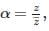then |α| is equal to :

Detailed Solution for Complex Numbers & Quadratic Equations- 2 - Question 1

α = |z/z¯|
z = x + iy
z¯ = x - iy
|α| = |z/z¯|
⇒ [(x2 + y2)1/2]/[[x2 + y2)1/2]
⇒ 1

Complex Numbers & Quadratic Equations- 2 - Question 2

### If z = x + iy ; x, y ∈ R then :

Complex Numbers & Quadratic Equations- 2 - Question 3

### The smallest positive integer n for which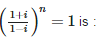Complex Numbers & Quadratic Equations- 2 - Question 4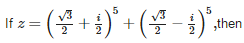Detailed Solution for Complex Numbers & Quadratic Equations- 2 - Question 4

z1 = (√3/2 + i/2), z2 = (√3 - i/2)
(z1)3 = (√3/2 + i/2)2
= 3/4 - 1/4 + √3i/2
= 1/2 + √3i/2
(z3)3 = (z1)2 * z
= (1/2 + √3i/2) * (√3/2 + i/2)
= √3/4 + i/4 + 3i/4 - √3/4
= i
(z2)2 = (√3/2 - i/2)2
= 3/4 - 1/4 - √3i/2
= 1/2 - √3i/2
(z2)3 = (z2)2 * z2
= (1/2 - √3i/2) * (√3/2 - i/2)
= √3/4 - i/4 - 3i/4 - √3/4
= -i
(z2)5 = (z2)3 * (z2)2
= (-i) * (1/2 - √3i/2)
= -i/2 + √3/2
z = (z1)5 + (z2)5
= i/2 - √3/2 - i/2 + √3/2
= 0
So Im(z) = 0

Complex Numbers & Quadratic Equations- 2 - Question 5

If the cube roots of unity are 1, ω,ω2, then roots of the equation (x−1)3+8 = 0 are :

Detailed Solution for Complex Numbers & Quadratic Equations- 2 - Question 5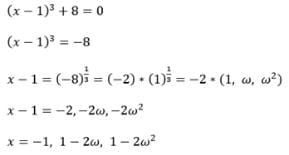Complex Numbers & Quadratic Equations- 2 - Question 6

Arg. (x) , x ∈ R and x < 0 is

Detailed Solution for Complex Numbers & Quadratic Equations- 2 - Question 6

z = x + iy
z = -x + 0i {-x > 0}
Principle arg(x) = π

Complex Numbers & Quadratic Equations- 2 - Question 7

The number of solutions of the equation Im (z2) = 0,|z| = 2 is

Detailed Solution for Complex Numbers & Quadratic Equations- 2 - Question 7

Let z = x + iy
Then z2 =  x2 + 2ixy - y2
And real(z2) = x2 - y2  = 0  …………...(1)
And |z| = √x2 + y2
|z| = 2
Hence √x2 + y2 = 2  ………..(2)
Or x2 +  y2 = 4 …….(3)
Solving (1) and (3) , we get x = ± √2 and
y = ± √2
It has 4 solutions.

Complex Numbers & Quadratic Equations- 2 - Question 8

Amp. (z−2−3i) = π/4 then locus of z is

Detailed Solution for Complex Numbers & Quadratic Equations- 2 - Question 8

z = x+iy
Hence ,(x−2) + i(y−3) = λ
Since the argument is π/4
​⇒ (x−2) = (y−3)
⇒ x − y = −1
⇒ y = x + 1
⇒ x - y + 1 = 0
Thus, slope is positive.

Complex Numbers & Quadratic Equations- 2 - Question 9

If z1 and zare non real complex numbers such that |z1| = |z2| and Arg(z1)+Arg(z2)= π, then z1 =

Detailed Solution for Complex Numbers & Quadratic Equations- 2 - Question 9

z1 = |z1(cosθ1 + i sinθ1)
z2 = |z2(cosθ2 + i sinθ2)|
Given that |z1| = |z2|
And arg(z1) + arg(z2) = pi
θ1 + θ2 = pi
⇒ θ1 = pi - θ2
Now, z1 = |z2|[cosθ(pi - θ2) + i sin(pi - θ2)]
= z1 = z2(-cosθ2 + i sinθ2)
= z1 = - |z2|(cosθ2 - i sinθ2)|
= z1 = - |z2|(cosθ2 - i sinθ2)
⇒ z1 = - z2(bar)

Complex Numbers & Quadratic Equations- 2 - Question 10

The complex numbers sinx + i cos2x and cosx – i sin2x are conjugate to each other, for

Complex Numbers & Quadratic Equations- 2 - Question 11

The value of  (-1 + √-3)2 + (-1 - √-3)2 is

Complex Numbers & Quadratic Equations- 2 - Question 12

If α is a complex a number such that α2+α+1 = 0 then α31 is

Detailed Solution for Complex Numbers & Quadratic Equations- 2 - Question 12

Since α3 +1=(α+1)(α2 −α+1), using the given information, we get α3 +1 = 0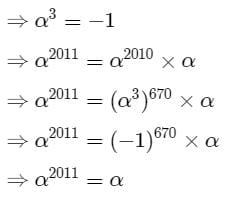Complex Numbers & Quadratic Equations- 2 - Question 13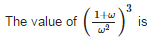Complex Numbers & Quadratic Equations- 2 - Question 14

If n is any integer, then in is

Complex Numbers & Quadratic Equations- 2 - Question 15

The points z = x + iy which satisfy the equation | z | = 1 lie on

Detailed Solution for Complex Numbers & Quadratic Equations- 2 - Question 15

z = x + iy
|z| = (x2 + y2)1/2
x2 + y2 = 1
Equation of circle with centre (0,0) and radius 1 unit.

Complex Numbers & Quadratic Equations- 2 - Question 16

The equation z2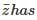Detailed Solution for Complex Numbers & Quadratic Equations- 2 - Question 16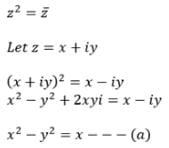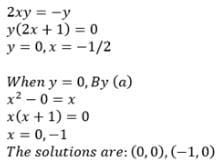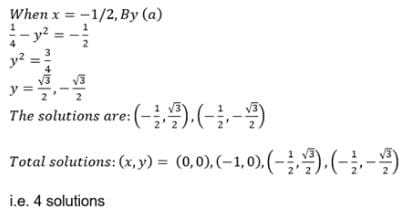Complex Numbers & Quadratic Equations- 2 - Question 17

The points of the complex plane given by the condition arg. (z) = (2n + 1) π, n ∈ I lie on

Complex Numbers & Quadratic Equations- 2 - Question 18

If z = x + yi ; x ,y ∈ R, then locus of the equation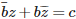, where c ∈ R and b ∈ C, b ≠ 0 are fixed, is

Detailed Solution for Complex Numbers & Quadratic Equations- 2 - Question 18

As b and c are linear constants ,independent of x and y, then by substituting them in the equation given, we get an equation linear in x and y. Thus, the given equation represents a straight line.

Complex Numbers & Quadratic Equations- 2 - Question 19

The complex number z which satisfies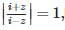lies on

Complex Numbers & Quadratic Equations- 2 - Question 20

(1+i)2n + (1−i)2n,n ∈ N is

Complex Numbers & Quadratic Equations- 2 - Question 21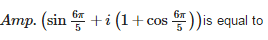Detailed Solution for Complex Numbers & Quadratic Equations- 2 - Question 21

sin(6π/5) + i(1+cos(6π/5))  or −sin(π/5) + i(1−cos(π/5)) lies in the second quadrant of complex plane hence its argument is given as
arg(x+iy) = π − tan-1 |y/x| (∀ x<0,y≥0)
= π−tan-1 |1−cos(π/5)/sin(π/5)|
= π−tan-1  |2sin2(π/10)/2sin(π/10)cos(π/10)|
= π−tan-1 |sin(π/10)/cos(π/10)|
= π − tan-1 |tan(π/10)|
= π−tan-1 (tan(π/10))  (∵ tan(π/10)>0)
= π−π/10(∵−π/2≤tan−1(x)≤π/2)
= 9π/10

Complex Numbers & Quadratic Equations- 2 - Question 22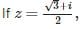then z69 is equal to :

Detailed Solution for Complex Numbers & Quadratic Equations- 2 - Question 22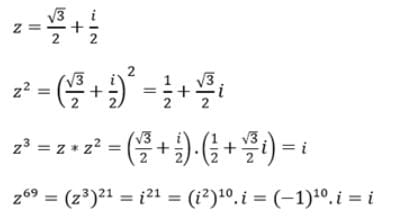Complex Numbers & Quadratic Equations- 2 - Question 23

If ω is a cube root of unity, then the linear factors of x3+y3 in complex numbers are

Complex Numbers & Quadratic Equations- 2 - Question 24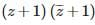can be expressed as

Detailed Solution for Complex Numbers & Quadratic Equations- 2 - Question 24

(z+1) (z(bar)+1)
((x+1)+iy) ((x+1)-iy)
= (x+1)2 + y2
= |(x+1)2 + iy2|
= |(x+iy) + 1|2
= |z+1|2

Complex Numbers & Quadratic Equations- 2 - Question 25

If α,β are non-real cube roots of unity then αβ + α55 equals

## Mathematics (Maths) Class 11

157 videos|210 docs|132 tests(Scan QR code)